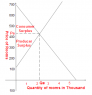# Understanding demand and supply curves

dpa
Hi everyone, I am having hardtime understanding this problem.
I have two functions:
QD = 5600 – 8P
QS = 500 + 4P

Why is the graph like the one attached and not the normal mathematical graph where supply curve starts from y=(0,-125)?
Do ignore the consumer surplus and producer surplus part. That is irrelevant.
How (and why) do we proceed in this way in our calculations?

#### Attachments

•asdasd.PNG
4.6 KB · Views: 575

alan2
P is the dependent variable in the graph. Rearrange the two equations and it will make sense (or reflect the graph about y=x). This is the one really annoying thing about an introduction to economics, you just get used to it. They always consider quantity to be a function of price but interchange them when graphing.

dpa
Suppose I rearrange the equations,
then P=700-0.125QD [which is fine without reflection and same as QD=5600-QD]
But, next P=0.25QS-125 but the graph is for 0.25QS+125. Note the + and _ after QS. It works with reflection. but not normally. Is there a certain convention. I mean am I always supposed to reflect along y=x line for supply curve only?
But then suppose we had QS=-500+4P. Would its y-intercept be (0,-125)?

alan2
Sorry, I didn't notice that. Algebra is still algebra, somebody made an error. The supply curve graphed is not the equation given. My guess is that they intended QS=4P-500 because the equation as written makes no sense. It implies that a producer would be willing to offer a supply of 500 at a price of zero which is absurd. So your equation is wrong, the graph given does not match the equation given, and the graph given does not match the alternative equation that I provided. They clearly intended that the producer would not offer anything until the price hit 125 but they messed up the whole thing. You should point this out. You have not lost your mind. :)

Staff Emeritus